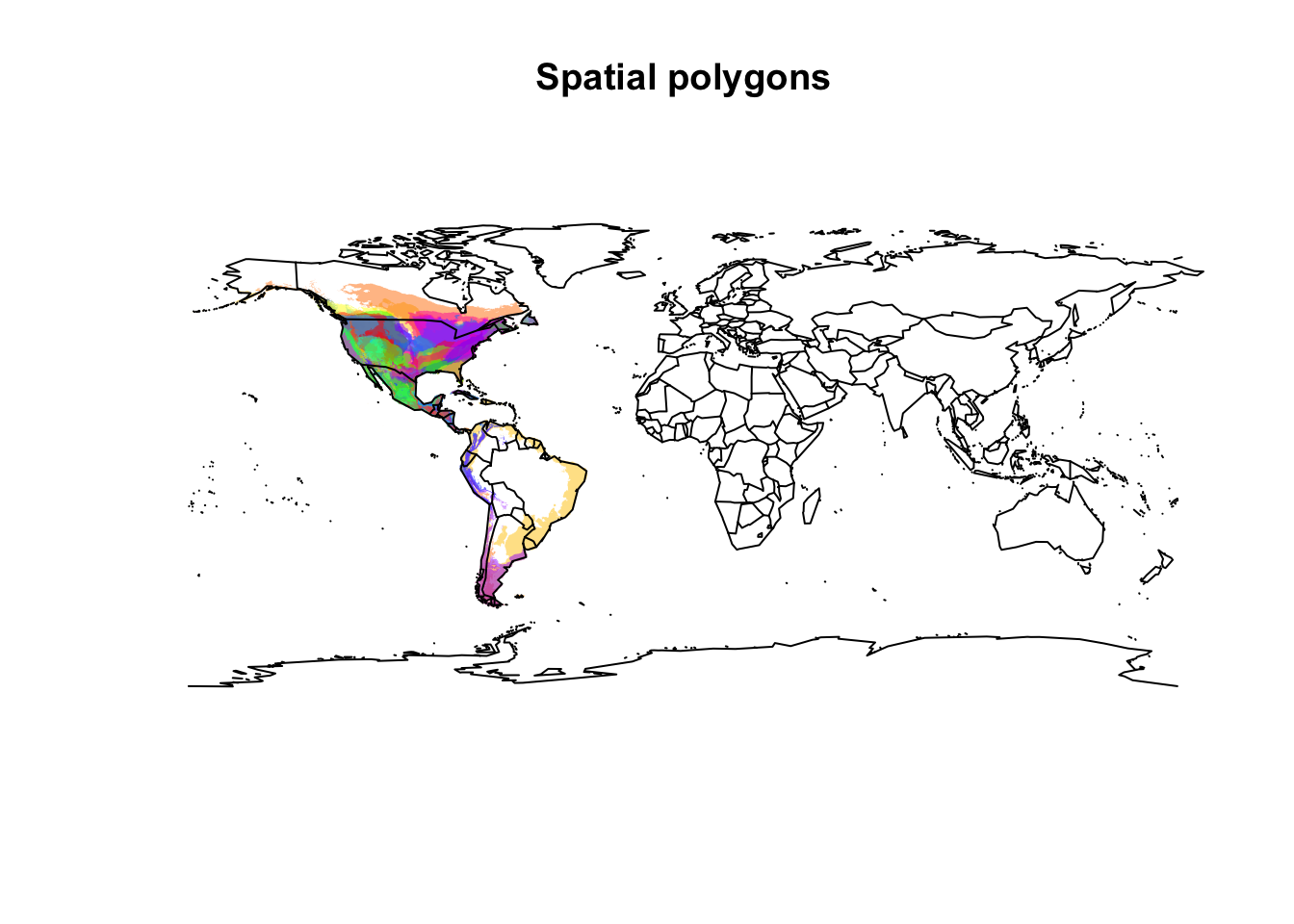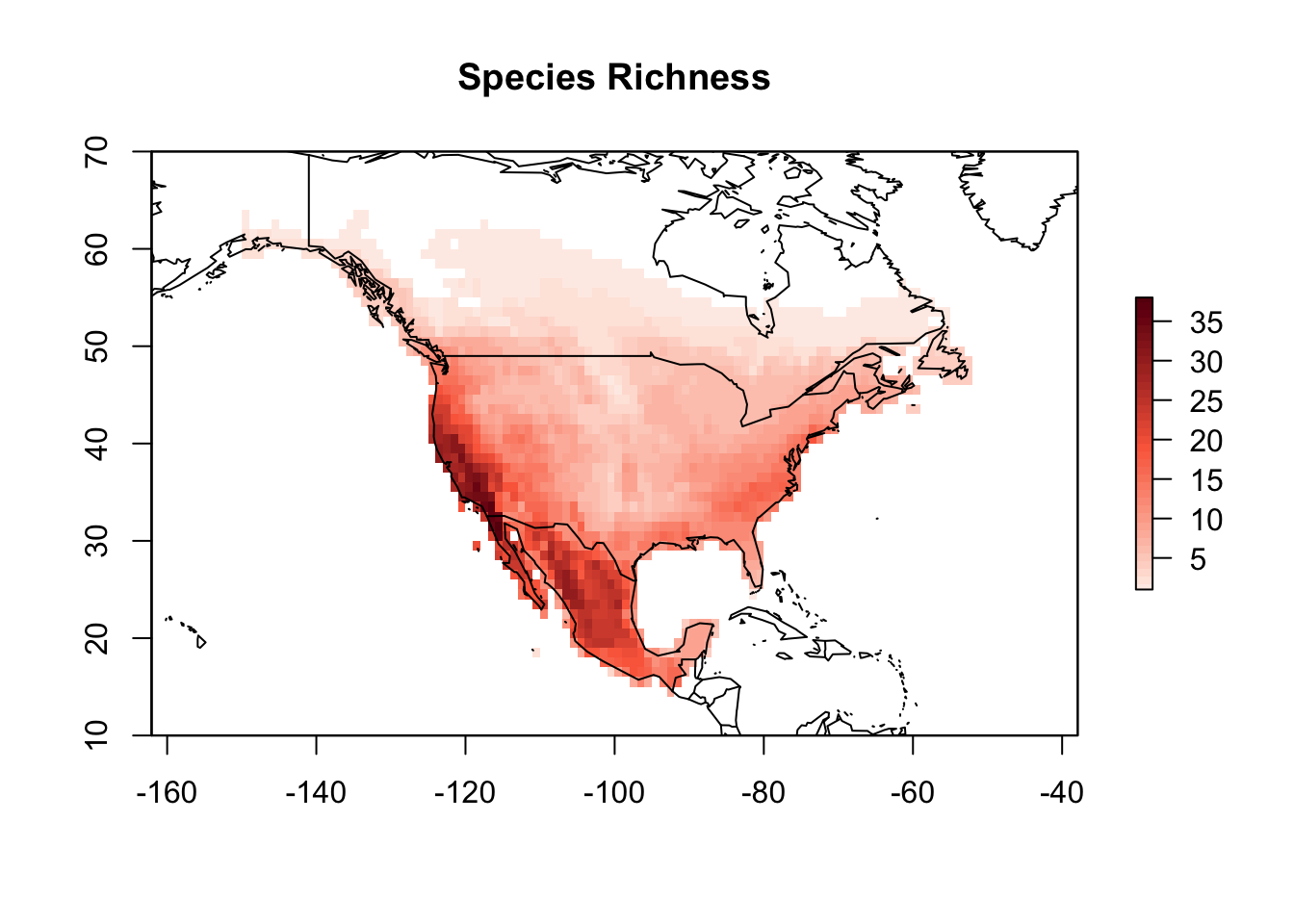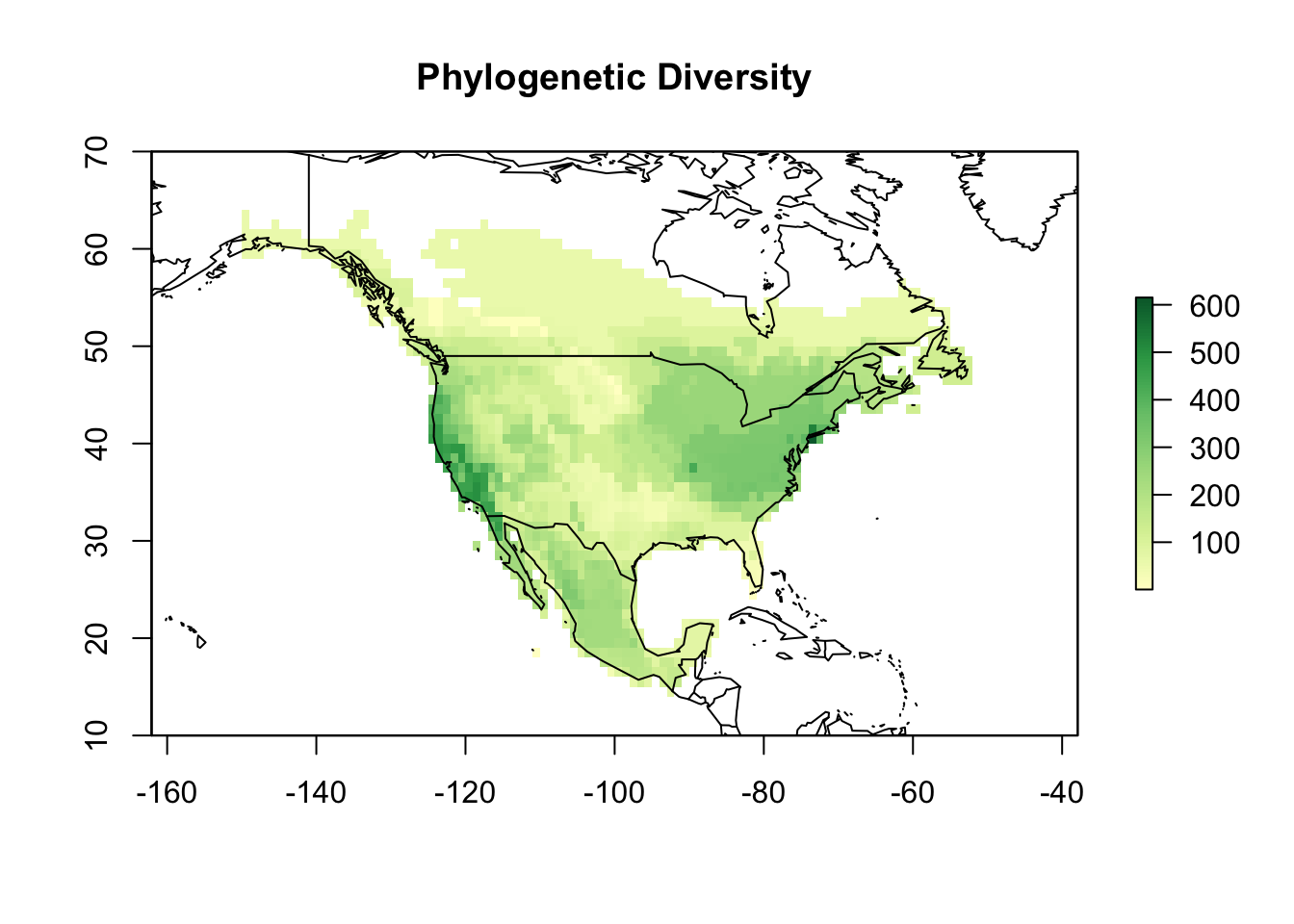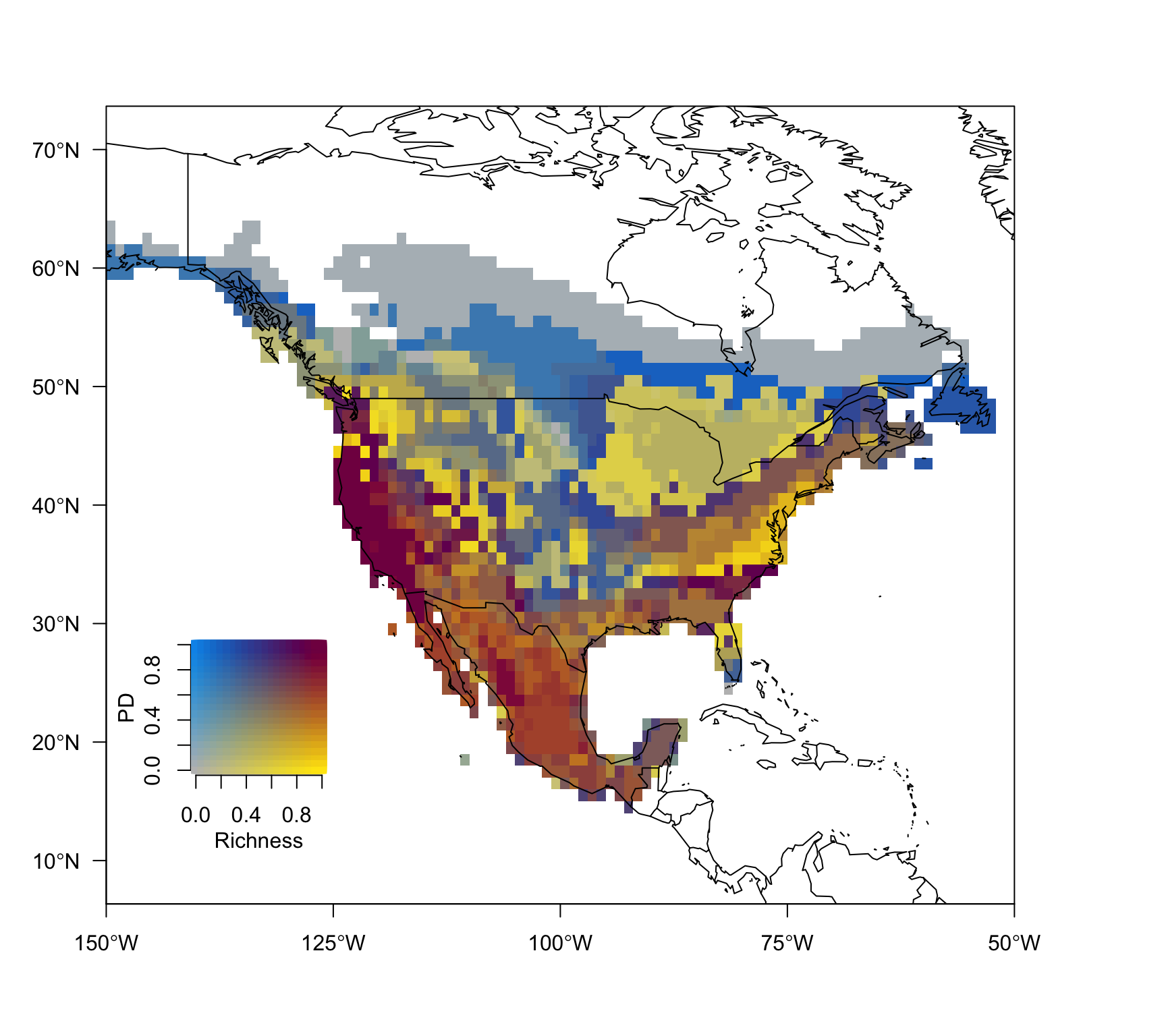# Mapping plants phylogenetic diversity

Retrieving plant species distributions from R has recently become quite easy using the BIEN R package, which facilitates the access to the Botanical Information and Ecology Network database. In addition to plant distributions, BIEN allow users to also obtain traits and phylogenetic plant data, making it a resourceful tool for macroecologists. Here I will show how to integrate BIEN and letsR to map phylogenetic diversity, using as an example the Pinus species of North America.

## Maping species richness

library(BIEN)
library(rgdal)
library(letsR)
library(maptools)
library(ggplot2)
library(ape)
library(classInt)
library(plotly)

To download the spatial distribution data of Pinus species, we have to first set up a directory where the shapefiles will be kept. For this case let’s just create a temporary folder.

temp_dir <- file.path(tempdir(), "BIEN_temp")

Now you can use the BIEN_ranges_genus function to download the shapefiles for each species of the genus that is available at the Botanical Information and Ecology Network database.

sp <- BIEN_ranges_genus("Pinus", temp_dir)

In this case we obtained the range of 84 species.

The code below can then be used to load every downloaded species shapefiles into a single SpatialPolygonsDataFrame object in R.

list_species <- unique(gsub("\\..*", "", list.files(temp_dir)))
shapes <- list()
for (i in 1:length(list_species)) {
shapes[[i]] <- rgdal::readOGR(dsn = temp_dir, layer = list_species[i],
verbose = FALSE)
}
all_shapes <- do.call(rbind, shapes)

Prior to be able use the functions in letsR, it is necessary to change the column name that indicate the species scientific names in the shapefile data. This transformation makes sure that the nomenclature used matches the same used by IUCN spatial data. This is important because IUCN shapefiles determine the standard format accepted by letsR functions. So, the name of the column should be either “binomial” or “sciname” (upper or lowercase).

colnames(all_shapes@data) <- "binomial"

To make a visual inspection of the data we just downloaded, we can plot the species spatial distributions to check the shapefiles.

colors <- rainbow(length(unique(all_shapes@data$binomial)), alpha = 0.5) position <- match(all_shapes@data$binomial,
unique(all_shapes@data$binomial)) colors <- colors[position] plot(all_shapes, col = colors, lty = 0, main = "Spatial polygons") map(add = TRUE)Once we have changed the species column name, we can directly use the function lets.presab. Notice, however, that the options presence, origin and seasonal cannot be used in this case, since this information is not available in the shapefiles. PAM <- lets.presab(all_shapes) Since we are only interested in North America for this example, we need to crop the PAM object to limit species distributions only to this continent, and remove species that only occur outside of it. data(wrld_simpl) # World map NoAme <- c("United States", "Canada", "Mexico") na <- wrld_simpl[wrld_simpl$NAME %in% NoAme, ]
PAM_na <- lets.pamcrop(PAM, na, remove.sp = TRUE)
plot(PAM_na, xlim = c(-150, -50), ylim = c(10, 70),
main = "Species Richness")## Maping phylogenetic diversity

Next step is to obtain the phylogeny. For this, we can use the function BIEN_phylogeny_complete, which will provide a more complete but less conservative phylogenetic hypothesis. For this example we will only download one unique tree for simplicity, but you may want to consider taking into account phylogenetic uncertainty in your own work, which in this case you would need to download several trees.

tree <- BIEN_phylogeny_complete(1)

Let’s use a simple measure of Phylogenetic Diversity (PD), determined as the sum of all co-occurring species branch lengths (Faith 1992).

Note that there are several metrics of phylogenetic diversity, the decision of which metric you should use will vary depending on the objectives of the research.

value <- which(tree$tip.label %in% PAM_na$Species_name)
sps <- tree$tip.label[value] names(tree$edge.length) <- tree$edge[, 2] branch_length <- tree$edge.length[as.character(value)]
sps2 <- PAM_na$Species_name[!(PAM_na$Species_name %in% sps)]

Note that 16 species are not in the phylogenetic tree. This is very common, and there are several ways to deal with this. To simplify the things here, I will just attribute the average branch length values of the genus to the species not considered in the tree. This is not an optimal method to deal with this (mainly at the genus level), and readers may consider more sophisticated approaches that will not be covered here now.

sps2_bran <- rep(mean(branch_length), length(sps2))

Now, we can then use the function lets.maplizer to map Pinus PD…

PD <- lets.maplizer(PAM_na, c(branch_length, sps2_bran),
c(sps, sps2),
func = sum,
ras = TRUE)

and plot it.

colors <- c('#ffffcc','#d9f0a3','#addd8e','#78c679','#31a354','#006837')
plot(PD$Raster, col = colorRampPalette(colors)(100), xlim = c(-150, -50), ylim = c(10, 70), main = "Phylogenetic Diversity") map(add = TRUE)As you can see in the graph below, species richness and phylogenetic diversity are highly correlated in this metric. rich <- rowSums(PAM_na$Presence_and_Absence_Matrix[, -c(1, 2)])
data <- data.frame("Richness" = rich, "PD" = PD$Matrix[, 3]) g <- ggplot(data, aes(Richness, PD)) g2 <- g + geom_point() + geom_smooth(method = 'lm',formula = y ~ x) ggplotly(g2) Based on this, it would be interesting to combine both species richness and phylogenetic diversity map into one unique map. This allow us to identify for example areas of high phylogenetic diversity given a low number of species richness and many other interesting patterns. For this we can use bivariate maps. This functions are not implemented in any package, but luckily Jose Hidasi has a post showing how to do them. The function below is the same function he provide in his post, with some very few modifications. # Load: colmat <- function(nquantiles = 10, upperleft = rgb(0,150,235, maxColorValue = 255), upperright = rgb(130,0,80, maxColorValue = 255), bottomleft = "grey", bottomright = rgb(255,230,15, maxColorValue = 255), xlab = "x label", ylab = "y label", plot = FALSE, ...) { my.data <- seq(0,1,.01) my.class <- classIntervals(my.data, n = nquantiles, style = "quantile") my.pal.1 <- findColours(my.class, c(upperleft, bottomleft)) my.pal.2 <- findColours(my.class, c(upperright, bottomright)) col.matrix <- matrix(nrow = 101, ncol = 101, NA) for (i in 1:101) { my.col <- c(paste(my.pal.1[i]), paste(my.pal.2[i])) col.matrix[102 - i,] <- findColours(my.class, my.col) } if (plot) { plot(c(1, 1), pch = 19, col = my.pal.1, cex = 0.5, xli = c(0,1), ylim = c(0,1), frame.plot = FALSE, xlab = xlab, ylab = ylab, cex.lab = 1.3) for (i in 1:101) { col.temp <- col.matrix[i - 1,] points(my.data, rep((i - 1) / 100, 101), pch = 15, col = col.temp, cex = 1) } } seqs <- seq(0, 100, (100 / nquantiles)) seqs <- 1 col.matrix <- col.matrix[c(seqs), c(seqs)] } # Plot bivariate map bivariate.map <- function(rasterx, rastery, colormatrix = col.matrix, nquantiles = 10) { quanmean <- getValues(rasterx) temp <- data.frame(quanmean, quantile = rep(NA, length(quanmean))) brks <- with(temp, quantile(temp, na.rm = TRUE, probs = c(seq(0, 1, 1 / nquantiles)))) while (any(duplicated(brks))) { brks <- ifelse(duplicated(brks), brks + (0.01 * min(brks[brks > 0])), brks) } r1 <- within(temp, quantile <- cut(quanmean, breaks = brks, labels = 2:length(brks), include.lowest = TRUE)) quantr <- data.frame(r1[,2]) quanvar <- getValues(rastery) temp <- data.frame(quanvar, quantile = rep(NA, length(quanvar))) brks <- with(temp, quantile(temp,na.rm = TRUE, probs = c(seq(0, 1, 1 / nquantiles)))) while (any(duplicated(brks))) { brks <- ifelse(duplicated(brks), brks + (0.01 * min(brks[brks > 0])), brks) } r2 <- within(temp, quantile <- cut(quanvar, breaks = brks, labels = 2:length(brks), include.lowest = TRUE)) quantr2 <- data.frame(r2[, 2]) as.numeric.factor <- function(x) {as.numeric(levels(x))[x]} col.matrix2 <- colormatrix cn <- unique(colormatrix) for (i in 1:length(col.matrix2)) { ifelse(is.na(col.matrix2[i]), col.matrix2[i] <- 1, col.matrix2[i] <- which(col.matrix2[i] == cn)) } cols <- numeric(length(quantr[, 1])) for (i in 1:length(quantr[, 1])) { a <- as.numeric.factor(quantr[i, 1]) b <- as.numeric.factor(quantr2[i, 1]) cols[i] <- as.numeric(col.matrix2[b, a]) } r <- rasterx r[1:length(r)] <- cols return(r) } Once you load the function above, we can use it to generate our maps. col.matrix <- colmat(nquantiles = 20) bivmap <- bivariate.map(rasterx = PAM_na$Richness_Raster,
rastery = PD\$Raster,
colormatrix = col.matrix,
nquantiles = 20)
plot(bivmap, frame.plot = FALSE, add = FALSE,
legend = FALSE, col = as.vector(col.matrix),
xlim = c(-150, -50), ylim = c(10, 70), axes = F)
xat <- seq(-150, -50, 25)
yat <- seq(-10, 70, 10)
xlab <- parse(text = degreeLabelsEW(xat))
ylab <- parse(text = degreeLabelsNS(yat))
axis(1, at = xat, labels = xlab, cex = .5)
axis(2, las = TRUE, at = yat, labels = ylab, cex = .5)

map(add = TRUE, fill = F)

par(new = T, fig = c(1.5, 3 ,2.3, 4) / 10)
colmat(nquantiles = 20, plot = TRUE)
mtext("Richness", 1, 2, font.lab = 2)
mtext("PD", 2, 2, font.lab = 2)From the map above we can infer for example that the US west coast has both the highest number of Pinus species richness and phylogenetic diversity values.

Many other things can be explored by integrating letsR and BIEN, and I hope that this example can be a good starting point for further explorations.

To cite letsR in publications use: Bruno Vilela and Fabricio Villalobos (2015). letsR: a new R package for data handling and analysis in macroecology. Methods in Ecology and Evolution. DOI: 10.1111/2041-210X.12401